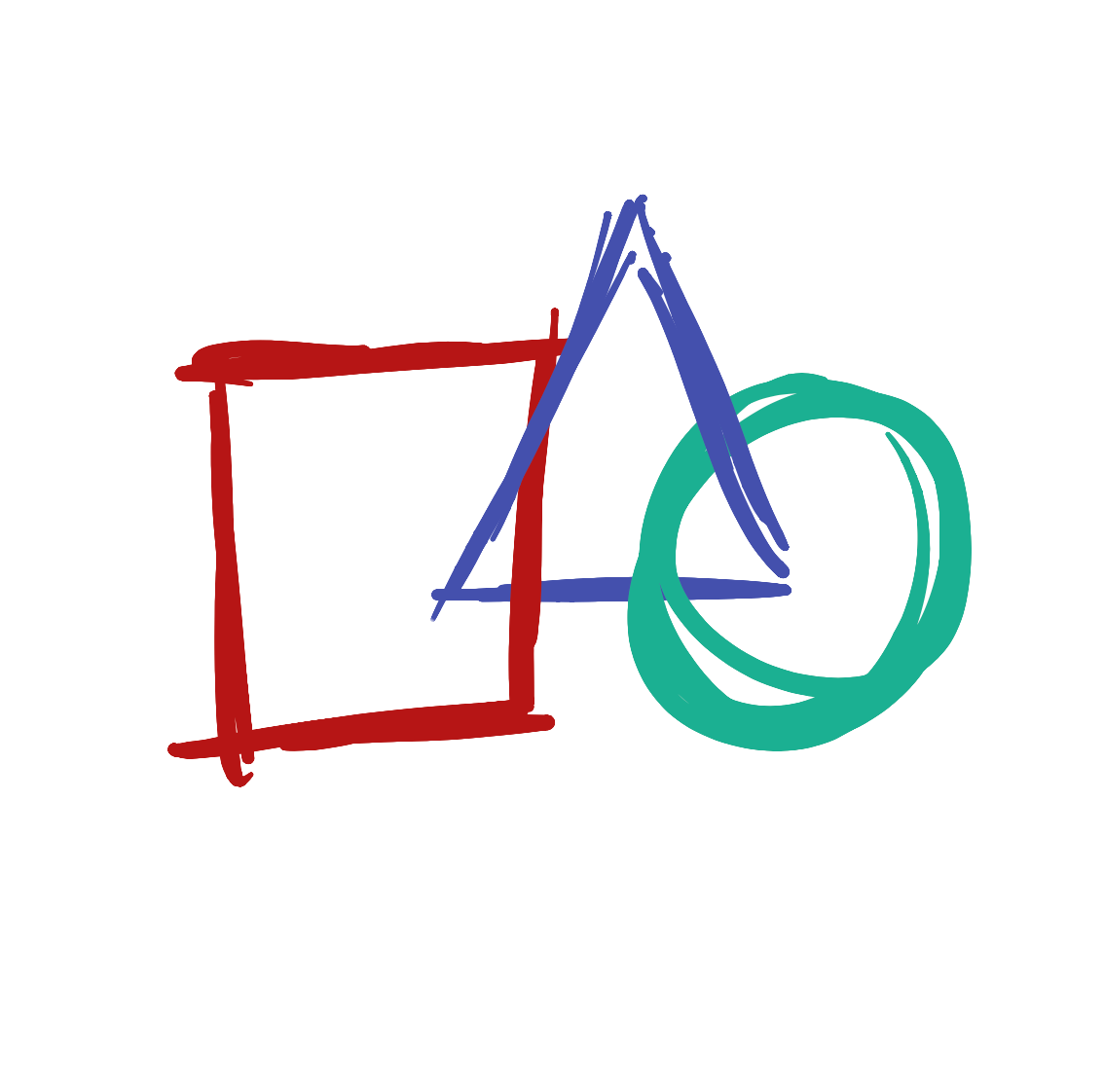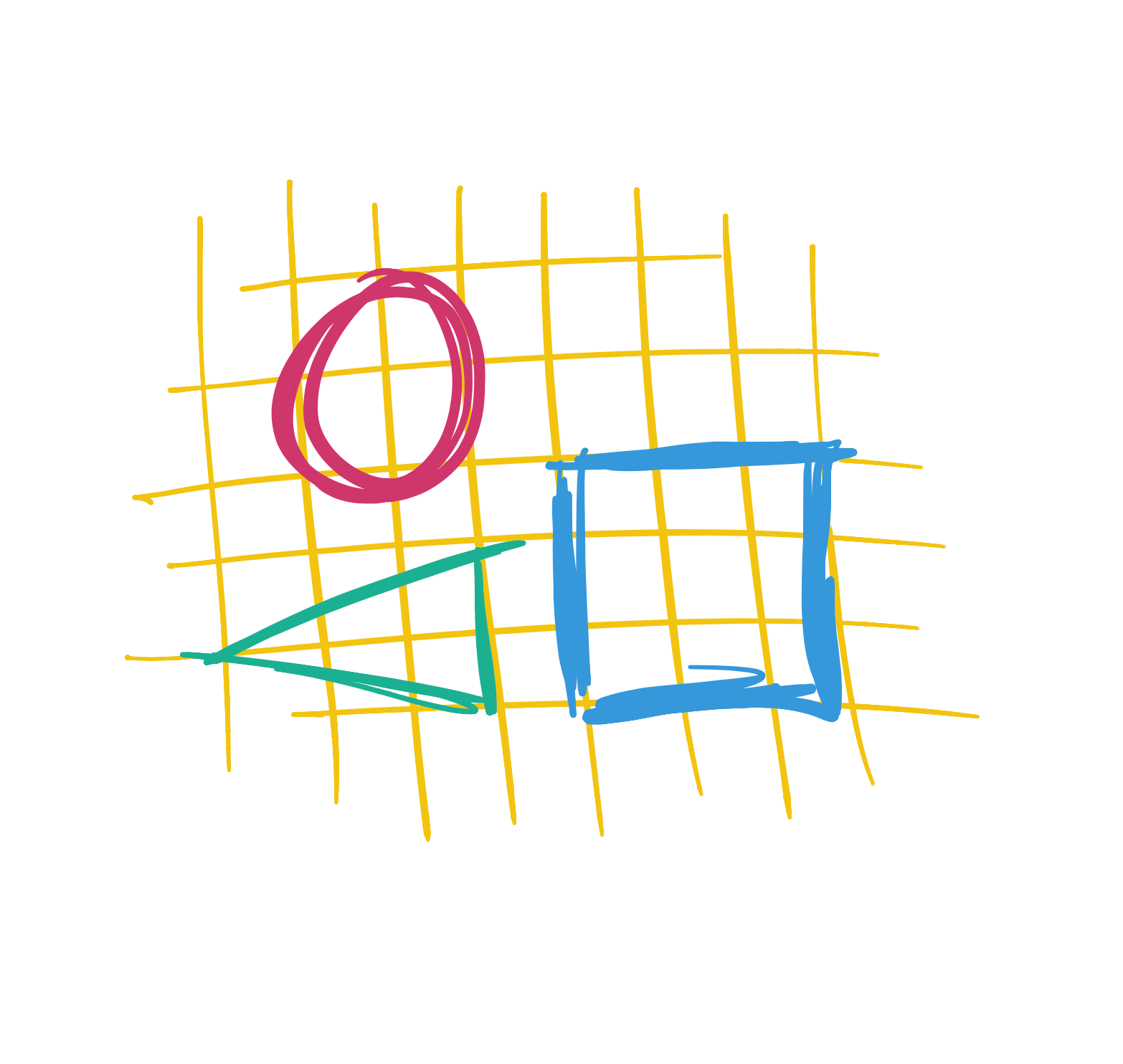# Drawing more shapesWe just drew a circle with the `ellipse` function, but there are so many other types of shapes we can draw.

ellipse()

Draws an ellipse (oval) to the screen

Learn More

## Rectangles and Squares

Let’s make a square! Press the ‘Backspace’ key until all of your code is removed, and then type the line below:

```rect(10,10,50,50);
```

Now press the Play button. You should see this on your screen:

This program looks a lot like our first program, but we replaced the `ellipse` function with the `rect` function. We have now told the computer we want to draw a rectangle instead of a circle.

rect()

Draws a rectangle to the screen

Learn More

## Lines

Let’s try a different function. Replace the `rect` function with `line`.

Your program should look like this:

```line(10,10,50,50);
```

Now press the Play button. You should see this on your screen:

line()

Draws a line (a direct path between two points) to the screen

Learn More

## Triangles

Let’s try drawing a triangle! Press the ‘Enter’ key, then type the line below:

triangle(50,50,20,90,80,90);

Your program should look like this:

```line(10,10,50,50);
triangle(50,50,20,90,80,90);
```

Now press the Play button. You should see this on your screen:

We just did a couple of interesting new things! All of the functions we have written until now have four numbers, but the triangle function has six! Some functions - like triangle - need more information than others.

triangle()

A triangle is a plane created by connecting three points

Learn More

The other thing we just did is we wrote a program that has two instructions. When we run the program, the computer will first create a line and then create a triangle. This is why we need to type the `;` semicolon at the end of each instruction, so the computer knows when to start the next instruction. You would think it could figure this out every time you type a new line, but computers aren’t very smart.

We’ve tried a bunch of functions, but each one of them has these weird numbers like `(10,10,10,10)` next to it. What do those mean? Let’s look at that next.

Next lesson Placing things on the screen